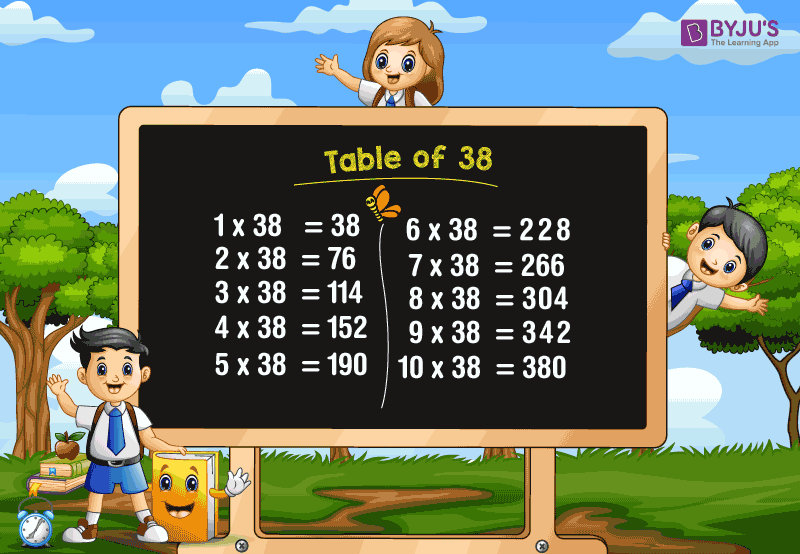# Table of 38

Table of 38 is essential for students as well as the candidates who are preparing for the competitive examinations. Learning the table of 38 will help students to solve the complicated problems, and it reduces the time while solving the problems. Here, we will learn the multiplication table of 38 with the help of a chart and will also learn how to read the 38 times table.

### Download Table of 38 Here## 38 Times Table

The table of 38 is the multiples of 38. The 38 times table can be obtained by multiplying 38 with the various whole numbers. Also, if we add the number 38 repeatedly, we get the table of 38, as multiplication is the repeated addition process.

For instance, 38 times 4 is 152.

(i.e.) 38 × 4 = 152

Similarly, if we add 38 for 4 times, we get the same result.

(i.e.) 38+38+38+38 = 152.

Also, check tables from 1 to 100 for more details.

## Multiplication Table of 38

Learn the table of 38 with the help of the chart given below. The chart provides the multiplication of 38 with numbers from 1 to 20.

 38 × 1 = 38 38 × 2 = 76 38 × 3 = 114 38 × 4 = 152 38 × 5 = 190 38 × 6 = 228 38 × 7 = 266 38 × 8 = 304 38 × 9 = 342 38 × 10 = 380 38 × 11 = 418 38 × 12 = 456 38 × 13 = 494 38 × 14 = 532 38 × 15 = 570 38 × 16 = 608 38 × 17 = 646 38 × 18 = 684 38 × 19 = 722 38 × 20 = 760

### How to Read 38 Times Table?

Read the table of 38 as follows:

• One time thirty-eight is 38
• Two times thirty-eight is 76
• Three times thirty-eight is 114
• Four times thirty-eight is 152
• Five times thirty-eight is 190
• Six times thirty-eight is 228
• Seven times thirty-eight is 266
• Eight times thirty-eight is 304
• Nine times thirty-eight is 342
• Ten times thirty-eight is 380
• Eleven times thirty-eight is 418
• Twelve times thirty-eight is 456
• Thirteen times thirty-eight is 494
• Fourteen times thirty-eight is 532
• Fifteen times thirty-eight is 570.

Get More Maths Tables:

## Frequently Asked Questions on Table of 38

### 1. What is the table of 38?

The table of 38, also called the 38 times table, is the multiplication of 38 with the different whole numbers. The 38 times table can be obtained by repeatedly adding the number 38.

### 2. What is the value of 7 times 38?

7 times 38 is 266. (i.e.) 7 × 38 = 266.

### 3. What are the first 5 multiples of 38?

The first five multiples of 38 are 38, 76, 114, 152, 190.

### 4. What is the cost of 5 pens, if each pen costs Rs. 38?

The cost of one pen is Rs. 38.
Therefore, the cost of 5 pens = 5 × 38 = 190.
Hence, the cost of 5 pens is Rs.190.

### 5. Find the value of x, if 38x=418.

The value of x is 11.
Explanation: 38x= 418
x = 418/38
x =11.